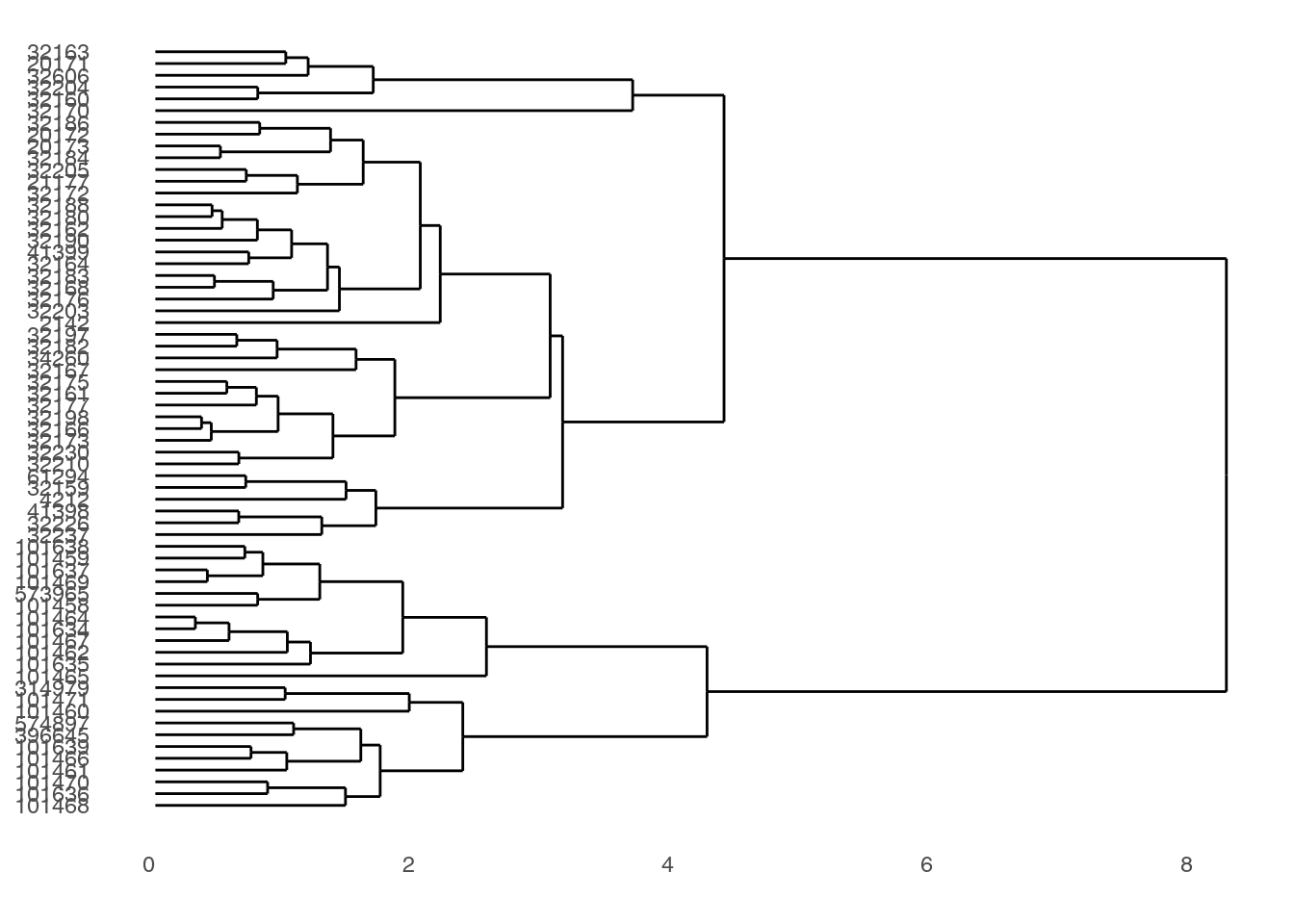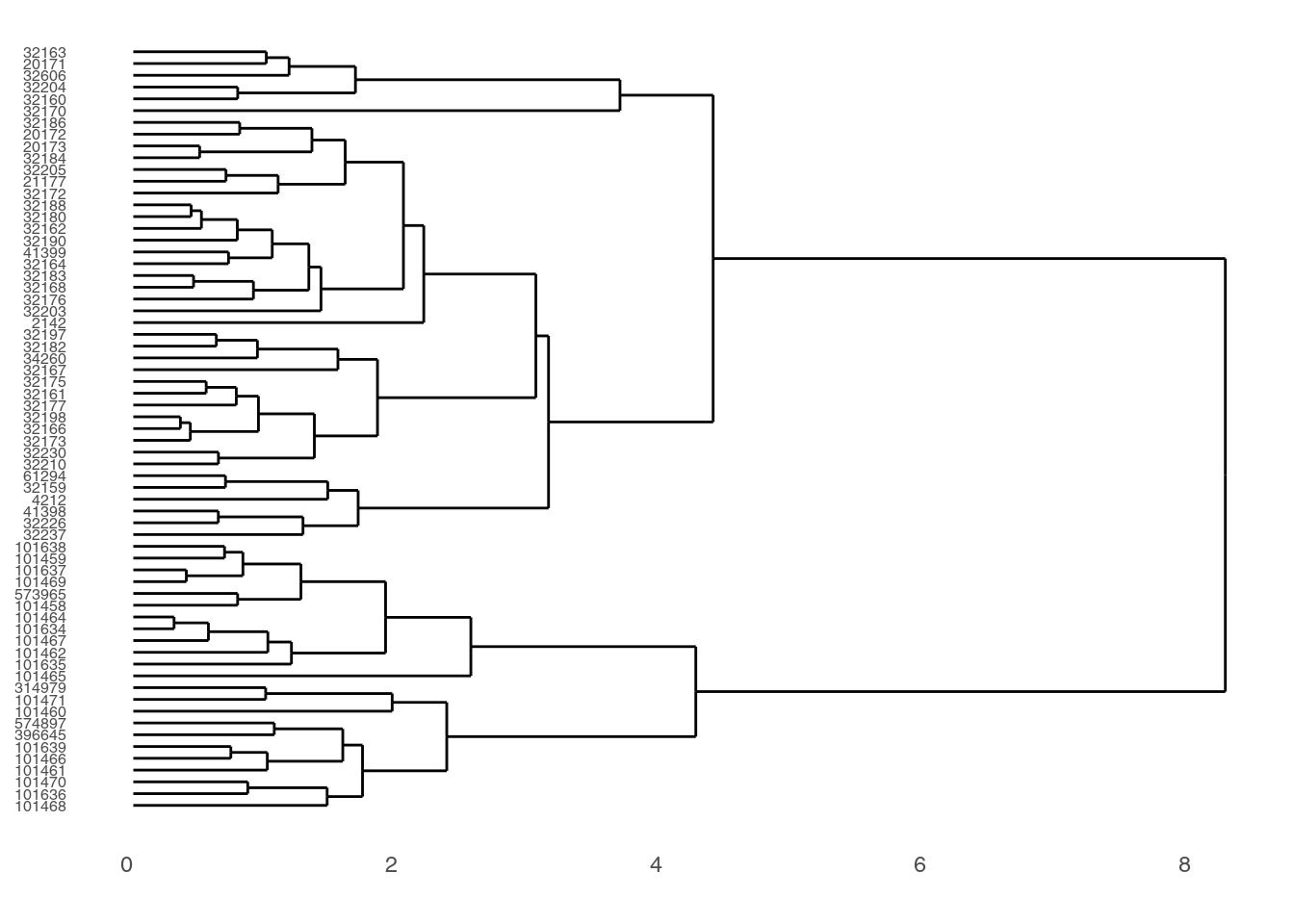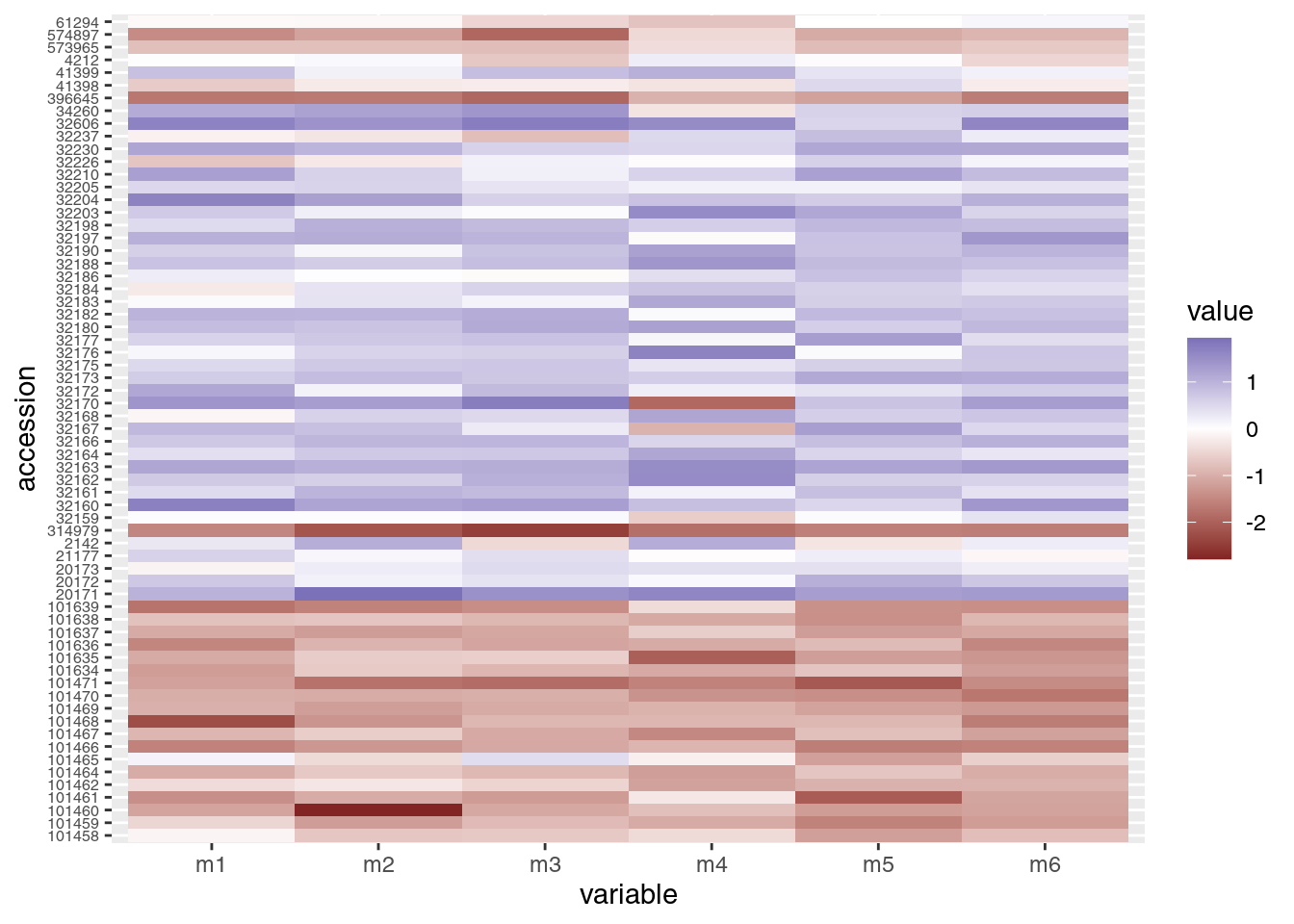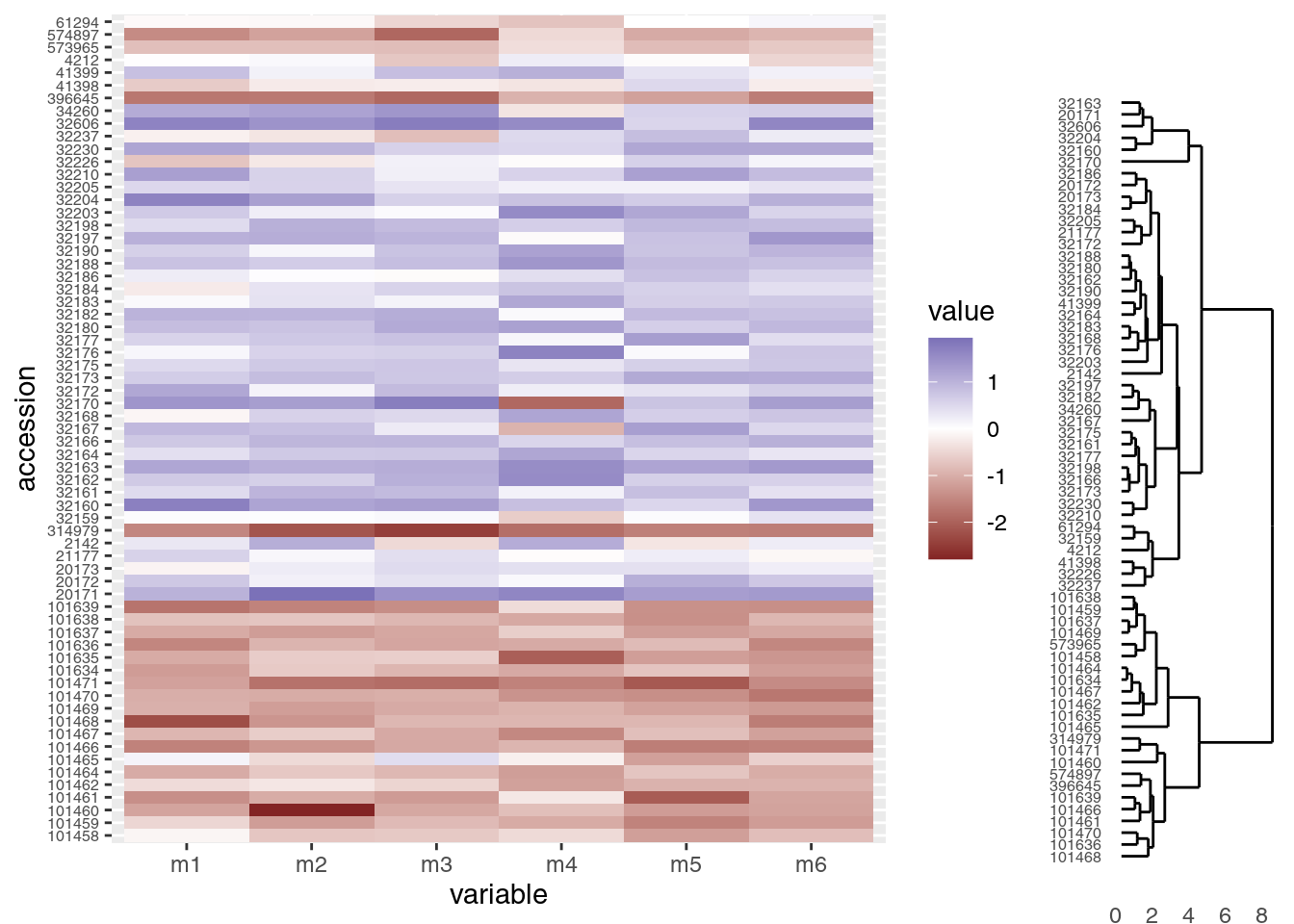Want to combine the visualization of quantitative data with clustering algorithms? Unsatisfied with the options provided by the base R packages? In this hands-on workshop, we’ll use the ggendro package for R to make publication-quality graphics.

#### Learning objectives

1. Run clustering analysis and display dedrogram
2. Visualize data in a heatmap
3. Use grid package to create multi-plot figures

## Getting started

Start by creating a new project in RStudio and creating two folders we’ll use to organize our efforts. The two folders should be data and output and will store…data and output.

dir.create("data")
dir.create("output")

We will also need a few R packages that are not included in the standard distribution of R. Those packages are ggplot2, ggdendro, reshape2, and grid and can be installed with the install.packages() function. Note these packages need only be installed once on your machine.

install.packages("ggplot2")
install.packages("ggdendro")
install.packages("reshape2")

Finally, download data file from https://jcoliver.github.io/learn-r/data/otter-mandible-data.csv or http://tinyurl.com/otter-data-csv (the latter just re-directs to the former). These data are a subset of those used in a study on skull morphology and diet specialization in otters doi: 10.1371/journal.pone.0143236. Save this file in the data folder we just created.

## Data preparation

For any work that we do, we want to record all the steps we take, so instead of typing commands directly into the R console, we keep all our work in an R script. These scripts are just text files with R commands; by convention, we start the script with a brief bit of information about what the script does.

# Cluster & heatmap on otter data
# Jeff Oliver
# jcoliver@email.arizona.edu
# 2017-08-15

rm(list = ls()) # clean up the environment

################################################################################

library("ggplot2")
library("ggdendro")
library("reshape2")
##
## Attaching package: 'reshape2'
## The following object is masked from 'package:tidyr':
##
##     smiths
library("grid")

stringsAsFactors = TRUE)

If we look at the first few rows of data, we see the first three columns have information about the specimen from which the measurements were taken, while columns 4-9 have data for six mandible measurements (m1 - m6):

head(otter)
##      species museum accession     m1     m2     m3     m4     m5     m6
## 1 A. cinerea   AMNH    101458 15.100 27.790 21.885 13.010 10.500 61.635
## 2 A. cinerea   AMNH    101461 12.740 26.750 20.265 13.255  8.340 59.370
## 3 A. cinerea   AMNH    101466 12.425 25.915 20.735 12.300  9.430 56.270
## 4 A. cinerea   AMNH    101635 13.400 28.030 22.075 10.580 10.455 58.080
## 5 A. cinerea   AMNH    101459 14.400 26.160 21.385 12.100  9.600 58.635
## 6 A. cinerea   AMNH    101462 14.525 29.020 22.305 11.905 11.070 60.655

For our purposes, we only want to look at the data for two species, so we subset the data, including only those rows that have either “A. cinerea” or “L. canadensis” in the species column. Note, this does not alter the data in the original file we read into R; it only alters the data object otter currently in R’s memory.

two.species <- c("A. cinerea", "L. canadensis")
otter <- otter[otter$species %in% two.species, ] The last thing we need to do is scale the data variables (columns 4-9) so measurements have a mean value of 0 and a variance of 1. otter.scaled <- otter otter.scaled[, c(4:9)] <- scale(otter.scaled[, 4:9]) ## Clustering To make our figure, we will build the two plots (the cluster diagram and the heatmap) separately, then use the grid framework to put them together. We start by making the dendrogram (or cluster). # Run clustering otter.matrix <- as.matrix(otter.scaled[, -c(1:3)]) rownames(otter.matrix) <- otter.scaled$accession
otter.dendro <- as.dendrogram(hclust(d = dist(x = otter.matrix)))

# Create dendro
dendro.plot <- ggdendrogram(data = otter.dendro, rotate = TRUE)

# Preview the plot
print(dendro.plot)We can make those labels a bit smaller:

dendro.plot <- dendro.plot + theme(axis.text.y = element_text(size = 6))

# Preview the plot
print(dendro.plot)That’s it for the cluster image (we’ll make a few more changes later on).

## Heatmap

We need to start by transforming data to a “long” format, where each row only contains values for one measurement. For example, our data started in this format:

##      species museum accession         m1         m2
## 1 A. cinerea   AMNH    101458 -0.1274976 -0.7008434
## 2 A. cinerea   AMNH    101461 -1.4031387 -1.0374187

We want to transform it so there is only a single measurement in each row:

##      species museum accession variable      value
## 1 A. cinerea   AMNH    101458       m1 -0.1274976
## 2 A. cinerea   AMNH    101461       m1 -1.4031387
## 3 A. cinerea   AMNH    101458       m2 -0.7008434
## 4 A. cinerea   AMNH    101461       m2 -1.0374187

This new data has columns that have information about the specimen (the “species”, “museum”, and “accession” columns), but now has “variable” and “value” columns. Instead of one column for each mandible measurement, each row only has one measurement in the “value” column, and the measurement type is stored in the “variable” column.

We use the reshape2 package’s melt function to handle the data transformation:

# Heatmap
# Data wrangling
otter.long <- melt(otter.scaled, id = c("species", "museum", "accession"))

Now we can use that new data frame, otter.long to create our heatmap. In the ggplot package, we use the geom_tile layer for creating a heatmap. Given our prior experience with the y-axis labels being large, we will again use theme to make the accession numbers (the y-axis labels) a little smaller:

heatmap.plot <- ggplot(data = otter.long, aes(x = variable, y = accession)) +
geom_tile(aes(fill = value)) +
theme(axis.text.y = element_text(size = 6))

# Preview the heatmap
print(heatmap.plot)## Putting it all together

OK. We made a dendrogram. We made a heatmap. Now how to get them into the same figure? The grid package offers a way to combine separate plots.

grid.newpage()
print(heatmap.plot, vp = viewport(x = 0.4, y = 0.5, width = 0.8, height = 1.0))
print(dendro.plot, vp = viewport(x = 0.90, y = 0.445, width = 0.2, height = 1.0))OK, that’s a start, but there are a few things we need to address:

1. Notice the tips of the dendrogram are not in the same order as the y-axis of the heatmap. We’ll need to re-order the heatmap to match the structure of the clustering.
2. It would be nice to have the dendrogram and heatmap closer together, without a legend separating them.
3. The dendrogram should be vertically stretched so each tip lines up with a row in the heatmap plot.

### Re-order heatmap rows to match dendrogram

We’ll use the order of the tips in the dendrogram to re-order the rows in our heatmap. The order of those tips are stored in the otter.dendro object, and we can extract it with the order.dendro function:

otter.order <- order.dendrogram(otter.dendro)

And now for the fun part. By default, ggplot use the level order of the y-axis labels as the means of ordering the rows in the heatmap. That is, level 1 of the factor is plotted in the top row, level 2 is plotted in the second row, level 3 in the third row and so on. So we can dictate the order in which these rows are plotted by setting the order of the levels in the column we use for the y-axis labels in the heatmap. The data we used for the heatmap was the long-format, otter.long object, and the column we used for the y-axis is accession. Using the order of the dendrogram tips (we stored this above in the otter.order vector), we can re-level the otter.long$accession column to match the order of the tips in the trees. # Order the levels according to their position in the cluster otter.long$accession <- factor(x = otter.long$accession, levels = otter.scaled$accession[otter.order],
ordered = TRUE)

At this point, we need to re-create the heatmap, because the underlying data (otter.long) has changed:

# Heatmap
heatmap.plot <- ggplot(data = otter.long, aes(x = variable, y = accession)) +
geom_tile(aes(fill = value)) +
print(heatmap.plot)